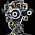10/19/2011

Incremental K-means matlab source code

Created Date : 2011.10.
Language : Matlab 2010
Tool : -
Library & Utilized :-
Reference :An Incremental K-means algorithm(D.T. Pham, S.S. Dimov and C.D. Nguyen)
Etc. :-

I made Incremental K-means algorithm as matlab source code.
I made the code base on above table which is introduced in the paper.
The incremental K-means is similar to K-means but the different point is number of cluster class is increasing. but we have to set the maximum number.

Below figure is result of clustering. the sample data is normal vector of the 3D point that is acquied by bumblebee.
I think the K-means algorithm is sensitive to error or outlier data. because the algorithm use euclidean distance. and The result of clustering is different every time because the initial position of the class is selected randomly.

I hope my code help to your problem and study.
thank you.

<source code>
Clustering result of the sample datas.

enlarge image of the above figure

1.The source code link is not working. Can you please update the link?

1.I am Sorry.
2.